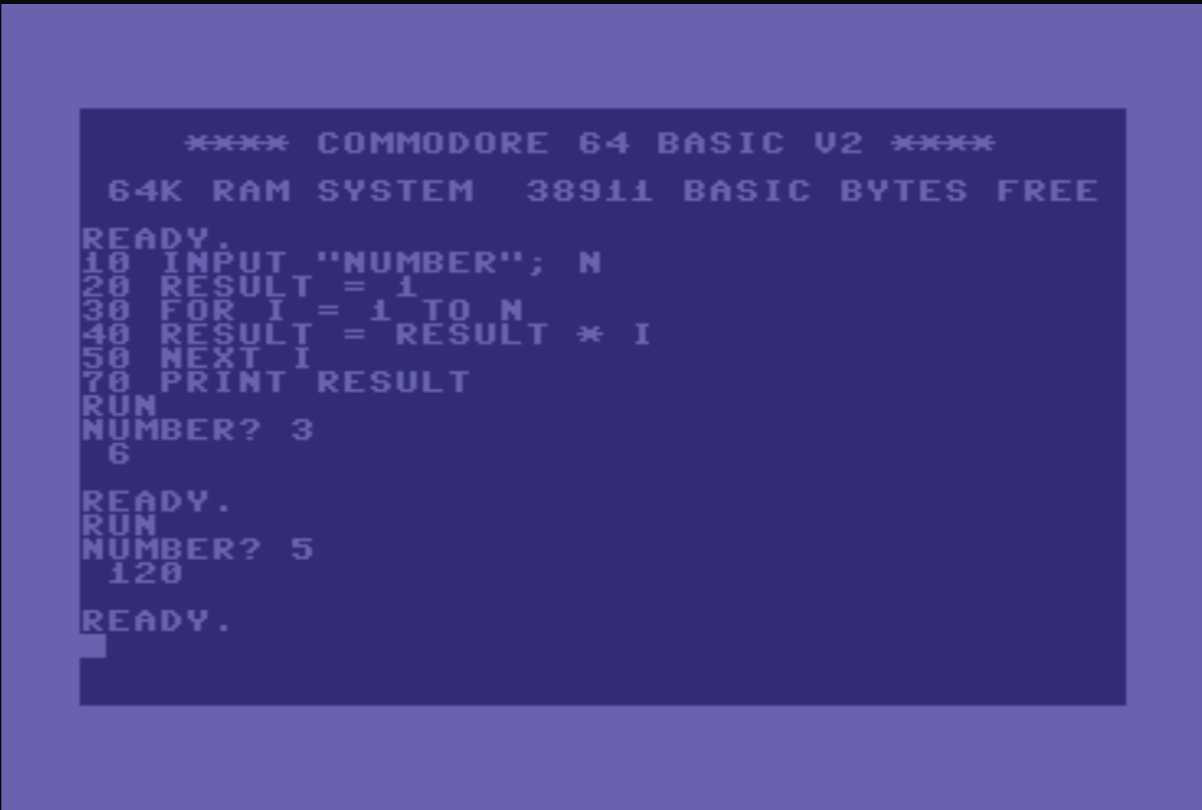When I was a tech writer, almost every time a developer interviewed me, they would inevitably ask me to whiteboard how to calculate the factorial of a number. When I moved to Northern California in 2008 and started interviewing at various Bay Area tech firms, I was seriously asked to do this exact problem at least twelve times in interviews with three companies.

I thought of this recently after reading this, and thinking of the smart-aleck answers I never did give during interviews, like writing it in PostScript or COBOL.

(BTW, a factorial is the product of all positive integers between 1 and n. It’s denoted as n! So 4! = 1 * 2 * 3 * 4 = 24.)

## Iteratively

The simple/obvious answer is doing it iteratively. In C:

``````int factorial(int n)
{
int i;
int result=1;
for (i=1; i<=n; i++){
result = result * i;
}
return result;
}
``````

Extra credit:

• That should fail if you called it with a zero or a negative number.
• There isn’t a `main()` that asks for a number and prints the result in a pretty way.
• If you want to be cute, use the assignment operator `result *= i` instead of `result = result * i` and save nine keystrokes.

## Recursively

They won’t always expect this from a writer who doesn’t regularly code, but here’s the other way to do it:

``````int factorial(int n)
{
if (n == 0)
return 1;
return n * factorial(n - 1);
}
``````

You can make this way more incomprehensible with the ternary operator:

``````int factorial(int n)
{
return(n==1||n==0) ? 1 : n*(factorial(n-1));
}
``````

## …Other

### Scheme

I went to Indiana University at a time when the first three or four computer science classes were all taught in Scheme. I always wanted to whiteboard this as an answer:

``````(define (factorial n)
(cond ((equal? n 0) 1)
(else (* n (factorial (- n 1))))))
``````

Extra credit: do it with tail recursion.

### Liquid

This blog is in Jekyll, so maybe you’ve worked with Liquid templates before:

``````{% assign n = 20 %}
{% assign result = 1 %}
{% for i in (1..n) %}
{% assign result = result | times i %}
{% endfor %}
{{ result }}
``````

(Tip: if you have to put a Liquid template in a code block in Jekyll, look at the source of this page in GitHub. I’d explain, but delimiting the text in another example isn’t working.)

### REST API

APIs are the future (not speaking for my employer, of course) so maybe you want to use a REST API to do it:

``````curl http://api.mathjs.org/v4/?expr=5!
``````

(Why use cURL though? There’s a much better way.)

### Standard libraries

If they forget to qualify their question with “don’t use standard libraries” you can do this (Python):

``````import math

def factorial(x):
print (math.factorial(x))
``````

### Commodore BASIC 2.0

Let’s take it back to the beginning:### SQL

I was trying to figure this out, but my SQL days are long behind me. You could use a recursive common table expression to create a table of n rows with each row containing the result of the previous row multiplied by the row number… or something.

### PDF

This is the point in my research where I had to give up and walk away for a bit. But if you had the right PDF CLI library, you could write a shell script that creates a one-page blank PDF, then iteratively merges copies of that template PDF onto a result PDF and count the number of pages at completion. There’s some magic number limit of objects in the PDF specification so they still work on 32-bit machines – eight million or so – which means this would break just past the factorial of 10.

Actually, a lot of these would break after about 12, when you’d hit `INT_MAX`. There’s a way to do bigger numbers by creating an array of the number of digits you want to calculate, then writing a multiply function that does all of the carrying when each spot in the array goes past 9, etc. If you really want to push it, use a linked list instead of an array so you won’t waste any space. This probably won’t fit on one whiteboard.

### PostScript

Oh, here it is in PostScript (I don’t know PostScript anymore; I borrowed it from here…)

``````/factorial { 1 dict begin
/n exch def
n 0 eq {
1
}{
n n 1 sub factorial mul
} ifelse
end } def
``````

(The serious answer to all of this, if you’re a developer tasked with interviewing a tech writer, is to ask them about their problem-solving methodology, and how they figure things out when they don’t know the answer. People who have to write a code sample every other month won’t know off the top of their head how to reverse a linked list. They should be able to talk you through a difficult problem they had to solve once, though. It’s probably a cross-functional review, right? Test their soft skills, not their ability to memorize. Just my opinion.)